Hypothesis Tests 1

Jim Mahoney, October 2003

Overview

This is a summary of the material in chapters 8, 9, and 10 in our Understanding Statistics text, which describe the basic idea behind hypothesis tests as well as several specific tests based directly on the binomal, the normal distribution, and the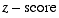that we've done in earlier chapters.

What's coming up next are several other more sophisticated tests, the Student's t-test, the Chi Square test, and the Anova test.  Each is appropriate for slightly different circumstances.  But first, the three situations we want to look at are
one sample tests of percentages (chap 8)
two sample tests of percentages (chap 9)
comparing means of large samples (chap 10)

The appropriate formulas in each case come from the same underlying principles, which I'm going to try to summarize here in one place.

The overall procedure for all these hypothesis tests is roughly the same, as described in the text near the end of chapter 8.  Typically you come up with a null hypothesis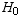which fits the model of one of of the tests, with a corresponding motivated hypothesis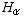which you are trying to show.  You then decide upon the data you'll collect, and a critical value of some parameter based on a chosen level of significance α.  The various kinds of tests have different formulas for these critical values, though the underlying idea is always based on a probability distrubtion.  Then you collect your data, compare your results with the critical value, and either reject the null hypothesis or not.  As discussed in the text and in class, there are two ways to come up with the wrong answer depending on whetheris really true or not; the probabilities of these Type-I and Type-II errors are named α and β.

Formulas

mean and standard deviation

Any random variable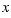with a probability distribution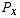has a mean μ and standard deviation σ given by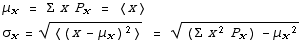We can add or subtract two such random variables to create a third, and express the new mean and standard deviation in terms of the old ones.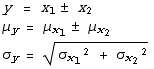These results assume that the two random variables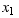and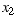are independent.  They follow directly from the definitions of mean and standard deviations and the fact that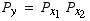.

binomial

A binomial random variablehas possible values 1 (success) and 0 (failure).
We call the probability of success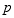and the probability of failure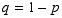.
In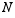trials the number of successes is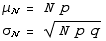binomial with N=1

Using a subscript "1" to emphasise that we're talking about one trial (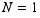), we have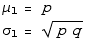percentage

Here's where it can get a bit confusing.
Often we would prefer to use the percentage (or fraction) of successes rather than the actual number of successes.  All we need do to get this percentage is divide by the number of trials N.  And since this is just a change of scale, the same division by N applies to the mean and standard deviation of this percentage.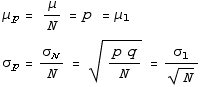difference of two percentages

Now we just apply the results from above.  If we do two surveys, with numbers of trials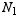and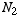respectively, and find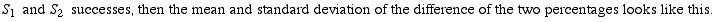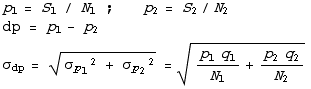standard deviation of the estimate of the mean

If we use a sample size ofto find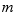, which estimates the true population mean, then a different sample will give a different.  Thusis also a random variable.  The mean value ofis μ, the true population mean, and the standard deviation is again smaller by the inverse of the square root of N, for the same reasons.  (However, whenis small, and we don't know the true population standard deviation, then its better to use the Student's t distribution than this σ and the normal distribution.)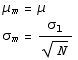standard deviation of the difference of means

Likewise, if we have two populations whose means we want to compare,  the difference of their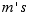estimated from samples of sizeandfollows the same kind of logic.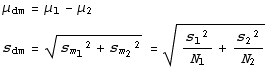z-score

For all of these, we can find probabilities by looking up the appropriate values for a normal distribution.  To do this from the table in appendix D-4, we convert to a standard normal distribution z, in which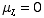and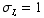.  Remember that this is only a reasonable approximation to the binomial if μ > 5 and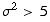.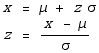in class 10/28

Created by Mathematica  (October 28, 2003)